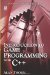# 10.2 Data Structures

## 10.2 Data Structures

A data structure is a collection of related variables. Like an array, data structures are a linear sequence of variables in memory; but unlike arrays, the variables can be of different types, stored one after the other. Taken as a whole, C++ treats any data structure as being a data type itself, so it is a means of defining new data types, as we shall see. Data structures are like the blueprints for data types and, like variables, they need to be declared. This can be achieved by using the struct keyword. The declaration for a data structure takes the following form:

`      struct name      {         Variable1;         Variable2;         Variable3;         [...]      }; `

### 10.2.1 Declaring Data Structures

For this example it'll be assumed that the data we are intending to store will be the scores for seven different exams each college student must take throughout the semester. The exact data to be recorded will be the student ID, which is unique to each student, and the score for each of the seven exams taken. An integer variable is used to store the student ID, and an array seven integer variables is declared to store the exam result. Together, these are stored in a data structure called StudentRecord. It will be the blueprint record for every student in the course. Consider the following sample program:

`      #include <iostream>      struct StudentRecord      {         int Student_ID;         int Exam_Results;      };      int main()      {         return 0;      } `
 Note Notice the struct itself is declared outside any function. The structure is not created in memory; it is a definition of a data type. It can be considered a template, or blueprint, upon which many different student records will later be created according to its structure. So right now, this program begins in main and then immediately ends; it does nothing.

### 10.2.2 Data Structures as Blueprints

As demonstrated previously, every data structure used in an application needs to be defined to the compiler. The data structure defines a new data type, and so it can be considered as the blueprint for variables declared as being of this type. Consider the following:

• int Variable;

Here, an integer variable is declared. This is called an instance, or an object.

• int Variable2;

Here, another integer is declared. Though this variable shares the same data type of the first (integer), it is still a different variable. So, this is a separate instance, or object.

• StudentRecord Record;

Here is another object. This variable has the data type of the recently created data structure, StudentRecord. Thus, data structures are similar to data types, and variables can be created from them in the same way as any other data type.

### 10.2.3 Data Structures and Members

As explained, data structures need to be defined to the compiler. A data structure defines a blueprint for objects that are declared as being of that type. Thus far, a sample data structure has been declared and contains two variables. The variables a data structure contains are called members. Again, the declaration is as follows:

`      struct StudentRecord      {         int Student_ID;        //First member         int Exam_Results;   //Second member      }; `

An object of data type StudentRecord can be declared as follows:

`      StudentRecord Record;      //So, in memory, Record = {      //                            int Student_ID = 0;      //                            int Exam_Results;      //                        } `

The question that remains, then is: How can the members of this object be accessed? To do this, the member operator (.) is used. Consider the following:

`      Record.Student_ID = 478;      Record.Exam_Results = 50;      Record.Exam_Results = 70;      // AND SO ON... `

### 10.2.4 Data Structures and Size

Recall that the size in memory of any data type or object can be determined using the sizeof function, as in the following example:

• int SizeOfInt = sizeof(int);

Similarly, the size of a data structure can be determined:

• int SizeOfRecord = sizeof(StudentRecord);Introduction to Game Programming with C++ (Wordware Game Developers Library)
ISBN: 1598220322
EAN: 2147483647
Year: 2007
Pages: 225
Authors: Alan Thorn

Similar book on Amazon

flylib.com © 2008-2017.
If you may any questions please contact us: flylib@qtcs.net